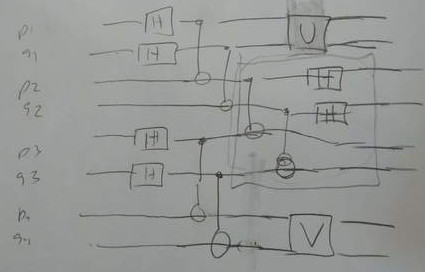# Quantum advantage with noisy shallow circuits in 3D

Sergey Bravyi, David Gosset, Robert Koenig, Marco Tomamichel

Main idea: There is a problem that 1D noiseless, 3D noisy constant depth quantum circuits solve but classical circuits need non-constant depth to solve.

The 1D problem uses the magic square game on 2 sets of $$n$$ qubit pairs where qubit pair $$j$$ from set 1 and $$k$$ from set 2 are inputs. The classical constant-depth circuit cannot achieve much above 8/9 chance of success.##### Things to clarify
• What is the X-type part of P(s)?

tags: NISQ